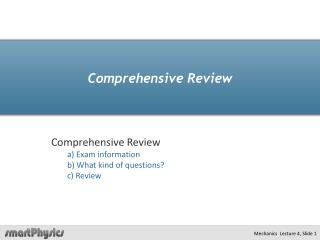# Comprehensive Review - PowerPoint PPT PresentationDownload PresentationComprehensive Review

Comprehensive ReviewDownload Presentation## Comprehensive Review

- - - - - - - - - - - - - - - - - - - - - - - - - - - E N D - - - - - - - - - - - - - - - - - - - - - - - - - - -
##### Presentation Transcript

1. Comprehensive Review Comprehensive Review a) Exam information b) What kind of questions? c) Review

2. Final Exam • In-class only!!! • Review the following material • homework problems. • video pre-lectures/textbook. • Lecture slides • Unit Main Points • Multiple choice…but show your work and justification. • Mostly Calculations…”step by step” • Some Conceptual questions…like checkpoint problems. • Bring calculators and up to ten sheets of notes. • It is best to prepare your own hand-written notes! • Derived Equations…(e.g. projectile motion)

3. General Topics • Kinematics • Description of Motion • Force • Dynamics-how objects change velocity • Energy • Kinetic and Potential • Conservation Laws • Momentum and Energy • Collisions • Elastic and In-elastic • Rotations • Torque/ Angular Momentum/Statics

4. Problem Solving Techniques • Visualize/Diagram • “Sketch” problem • Identify variables, input and what we are trying to solve • Free-body diagrams • Express in Mathematical Equations • Scalars-1d • Vectors-2d,3d Break into components • System of n-equations with n-unknowns • Use Mathematical tools to solve: • Quadratic Equation • Vector operations • Trigonometry • Conceptual Understanding • Does answer make sense?

5. Potential Problem Topics • Projectile Motion • Relative Motion - 2d • Uniform Circular Motion • Forces • Weight (near earth) • Gravitational (satellite) • Springs • Normal Force • Tension • Friction • Free-Body Diagrams • Work-Kinetic Energy • Potential Energy • Center of Mass • Conservation of Momentum • Collisions • In-elastic • Elastic • Rotations • Kinematics • Dynamics • Statics • Moment of Inertia • Torque • Angular Momentum

6. Relevant Formulae

7. Relevant Formulae

8. Kinematics

9. HyperphysicsMotion Displacement vs timet Velocity vs timet Acceleration vs timet

10. HyperphysicsMotion

11. 1d-Kinematic Equations for constant acceleration • Basic Equations to be used for 1d – kinematic problems. • Need to apply to each object separately sometimes with time offset • When acceleration changes from one constant value to another say a=0 The problem needs to be broken down into segments

12. Ballistic Projectile Motion Quantities Initial velocity speed,angle Maximum Height of trajectory, h=ymax “Hang Time” Time of Flight, tf Range of trajectory, D Height of trajectory at arbitrary x,t

13. Derived Projectile Trajectory Equations Maximum height Time of Flight (“Hang Time”) Range of trajectory Height of trajectory as f(t) , y(t) Height of trajectory as f(x), y(x)

14. Relative Motion in 2 Dimensions Direction w.r.t shoreline Speedrelative to shore

15. Uniform Circular Motion

16. Uniform Circular Motion • Constant speed in circular path • Acceleration directed toward center of circle • What is the magnitude of acceleration? • Proportional to: • Speed • time rate of change of angle or angular velocity v = wR

17. Dynamics

18. Inventory of Forces • Weight • Normal Force • Tension • Gravitational • Springs • …Friction

19. http://hyperphysics.phy-astr.gsu.edu/hbase/N2st.html#c1

20. Be careful what value you use for r !!! Should be distance between centers of mass of the two objects

21. m2 1) FBD N T m2 g f T m1 m1 m2g m1g

22. m2 1) FBD 2) SF=ma N T m2 g f T m1 m1 m2g N = m2g m1g m1g – T = m1a T – mm2g = m2a add m1g – mm2g = m1a + m2a m1g – mm2g a = m1 + m2

23. m2 1) FBD 2) SF=ma N T m2 g f T m1 m1 m2g m1g m1g – T = m1a m1g – mm2g T = m1g – m1a a = m1 + m2 T is smaller when a is bigger

24. Work-Kinetic Energy Theorem But again…!!! The work done by force F as it acts on an object that moves between positions r1 andr2is equal to the change in the object’s kinetic energy:

25. Energy Conservation Problems in general For systems with only conservative forces acting Emechanical is a constant

26. Determining Motion • Energy • Total Energy  Motion, Location • Work • Conservative forces • Motion from Energy conservation • Force • Unbalanced Forces  acceleration • (otherwise objects velocity is constant) • Determine Net Force acting on object • Use kinematic equations to determine resulting motion

27. Friction “It is what it has to be.”

28. Block

29. Work & Kinetic Energy

30. Example Problem

31. Potential Energy

32. Example Problems

33. Example: Pendulum h h Conserve Energy from initial to final position.

34. Gravitational Potential Problems • conservation of mechanical energy can be used to “easily” solve problems. • Define coordinates: where is U=0? as • Add potential energy from each source.

35. Collisions • Center of Mass • Conservation of Momentum • Inelastic collisions • Elastic Collisions • Impulse and Reference Frames Multiple particles, Solid Objects Isolated system, No external force Non-conservative internal force Conservative internal force Individual Particle changes momentum due to Force acting over a given duration Favg = DP/Dt

36. Systems of Particles

37. Example Problem

38. Collisions

39. Example Problem

40. Example Problem :

41. Impulse

42. |Favg | = |DP| /Dt = 2mv cosq /Dt

43. Rotations • Rotational Kinematics • Moment of Inertia • Torque • Rotational Dynamics • Rotational Statics • Angular Momentum Description of motion about a center of mass Resistance to changes in angular velocity Force applied at a lever arm resulting in angular acceleration Newton’s 2nd law for rotations How to ensure stability Vector Quantity describing object(s) rotation about an axis

44. Rotational Kinematics

45. Rotational Dynamics

46. Example Problem